# Simulation of corrugated plate heat exchanger for heat and flow analysis

Simulation of corrugated plate heat exchanger for heat and flow analysis

Muhammad AsifH. Aftab H.A. Syed M.A. Ali P.M. Muizz

Faculty of Mechanical Engineering, GIK Institute of Engineering Sciences and Technology, Topi, Swabi, KPK, 23640, Pakistan

Corresponding Author Email:
masif@giki.edu.pk
Page:
205-210
|
DOI:
https://doi.org/10.18280/ijht.350127
|
Accepted:
|
Published:
31 March 2017
| Citation

OPEN ACCESS

Abstract:

In this study thermal performance of corrugated plate heat exchanger (PHE) in single-phase flow is carried out using the ANSYS CFX software. Aim of this research is to develop a generalized Nusselt Number correlation for a commercial PHE configuration under single-phase flow for two different chevron angle plates, 30o/30o and 60o/60o. The steady state simulation is carried out for a Reynolds number range of 500 to 2500, and the Prandtl number range of 3.5 to 7.5 is maintained in the simulation. The results showed that Nusselt number is increased with increasing Reynolds number as well as by increasing the chevron angle. However, Prandtl number showed trivial impact on the Nusselt number.

Keywords:

Corrugated Plate Heat Exchanger, CFD Analysis, Heat and Flow Analysis, Nusselt Number Correlation, Modified Wilson Plot

1. Introduction

A heat exchanger is a device used for transferring heat from one fluid to another. The fluid may not be allowed to mix by separating them by a solid wall or they may be in direct contact. They are operated in numerous industries such as power generation, petroleum refineries, chemical and processing plants and HVACs. There are various types of heat exchangers available but our study pertains to the corrugated plate heat exchangers (CPHE). It comprises of multiple, thin plates, stacked upon each other. They have large surface areas and corrugations to make the flow turbulent for better heat transfer.

Numerous works has been done on Plate Heat Exchangers (PHEs) and their data related to thermal and hydraulic characterization are available in open literature. But there is a widespread discrepancy in these reported correlations and before commencing the present study, it was necessary to analyze the experimental facilities and procedure, data reduction methods, results and conclusions of some of the important past works. Shah and Sekulic  presented a comprehensive study on PHEs, including all characterization related to PHEs and single pass gasketed PHEs. The procedure of installing the PHEs in vertical configuration along with many other important aspects related to PHEs has been presented. In our study, the analysis technique for determining the core pressure drop and friction factor is used from the work described by Shah and Sekulic . Fernandez-Seara et al.  have provided a detailed review on the original Wilson Plot, number of its modifications and application to different heat exchangers over the last half century. The data reduction procedure for thermal performance adopted by Khan et al.  is used in the current study. This procedure requires the Reynolds Number (Re) to be kept constant on both hot and cold sides and assume the heat transfer coefficient on both sides to be same. Based on this data reduction procedure, two correlations have been proposed for two different chevron angle (β) configurations; 30°/30°, and 60°/60°. The Nusselt Number (Nu) is reported to increase with β and with Reynolds number. The friction factor (f) decreases with Re but increases with β . Although, Hashmi et al.  used similar data reduction method i.e. a Modified Wilson Plot Technique, but Nu correlation for the mixed plate configuration (β = 30°/60°) was reported only. Muley and Manglik  provided the comprehensive set of pressure drop correlations for PHEs using the Wilson Plot Technique . However, the effect of elevation pressure drop was ignored in friction factor calculations. Akturk et al.  presented Nu and f correlations only for the 30°/30° plate configuration. Although the trends presented is comparable with literature but the Nu versus Re plots does not represent a linear relation. Turk et al.  developed correlations to analyze the thermal and hydraulic performance of gasketed plate heat exchangers (GPHE) for a Reynolds’s number range of 500-5000. Quintero and Vera  presented exact solution for wall conduction effects in counter-flow parallel plate heat exchange in the laminar flow regime. The maldistribution of chevron angle, flow distribution and pressure drop in plate heat exchanger was analyzed by Kumar and Singh , while the impact of fouling on heat transfer performance was described by Hazmi et al. . The effect of various nano-fluids on heat transfer capacity of plate heat exchanger is presented by Doohan et al.  by energy and exergy analysis. Zhang et al.  predicted the correlations of friction factor and Nusselt number for capsule type plate heat exchanger. Whereas Wahiba et al.  carried out 3D CFD analysis of plate fin tube heat exchanger to predict the effect of inlet air flow maldistribution on design and thermal-hydraulic performance.

In the published literature of PHEs, references  and  have presented the detailed characterization of PHEs and experimental conditions. Others have missed several important geometric and experimental parameters. Moreover, they have used the same value of the corrugation depth (b) for both the configurations (30°/30°, and 60°/60°) during their experimentation, whereas in fact it varies in both configurations, being the highest for soft configuration and lowest for the hard plate configuration. In this study configurations of Nusselt number for the configurations (30°/30°, and 60°/60°) is developed for for a Reynolds’s number range of 500 to 2500 and the Prandtl number range of 3.5 to 7.5.

2. Design of Heat Exchanger

2.1 Details of corrugated plates

The corrugated plates are the main components of the simulation. The chevron angle, corrugation depth and corrugation pitch varied for the two types of plates used. The modular design of the heat exchanger allowed us to arrange the plates in the required configurations. The plate geometry used in the simulation is shown in Figure 1. The dimensional characteristics of chevron plate are shown in Table 1, while the geometric characteristics of chevron plate are shown in Table 2.

## 1.jpg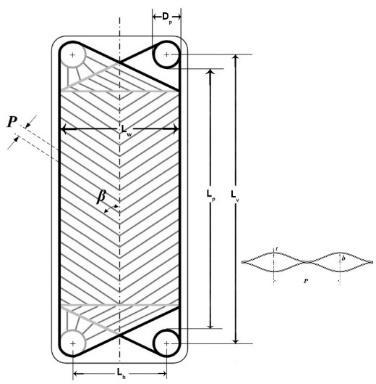Figure 1. Basic geometric characteristics of Chevron Plate

Table 1. Dimensional characteristics of Chevron Plate

 Geometric Characteristics Measurement Width of the plate, Lw (mm) 185 Height between centres of ports, Lv (mm) 565 Port diameter, Dp (mm) 43 Horizontal distance between centres of ports, Lh (mm) 125 Mean channel spacing, b (mm) 2.2 and 3.6a Plate thickness, t (mm) 0.5 Effective area of plate, A (m2) 0.095 Corrugation pitch, Pc (mm) 13.25 and 6.25b Surface enlargement factor, j 1.117

a for b = 60o/60o plate configurations

b for b = 30o/30o plate configurations

Table 2. Geometric characteristics of chevron plate

 Characteristics Description Chevron angle, β It is a measure of softness (small β, low thermal efficiency and pressure drop) and hardness (large β, high thermal efficiency and pressure drop) of thermal and hydraulic characteristics of plates. Some authors define π/2-β as the chevron angle. β typically varies from 20o to 65o. Surface enlargement factor, Ø Ratio between the developed area (based on corrugation pitch, Pc, and plate pitch, p) and the projected area (viz. LwxLp, where Lw = Lh + Dp and Lp = Lv – Dp) Corrugation depth or mean channel spacing, b b = p – t, the difference between plate pitch, p & plate thickness, t. Channel flow area, Ax Ax is the minimum flow area between the plates and is determined as the product of width and depth of the plate configurations (i.e. Ax = b x Lw) Channel hydraulic diameter, Dh Dh is defined as four times the ratio of minimum flow area to wetted perimeter,  $D_h=(2bL_w)/(b+L_{w∅})$  Since b << Lw, Dh is generally taken to be 2b/j.

In order to perform simulations, we need to build a CAD model. The measurements for the different geometric parameters mentioned in Table 1 and Table 2 are used to design a computer generated model using the PTC Creo Parametric software (version 3.0). The outer part for both the plates are same, only the corrugated portion of the plates is different for both the 30o and 60o plates, and therefore two different corrugations configurations are designed.

1.2.160o/60o chevron angle

The corrugations are sinusoidal and therefore a Cartesian coordinate system is set and the following equation is used to generate the curve in Creo Parametric:

The corrugations are sinusoidal and therefore a Cartesian coordinate system is set and the following equation is used to generate the curve in Creo Parametric:

x=6.25*t

y=1.1 sin⁡(360t)

z=0

This equation was repeated more than 62 times to get corrugated sinusoidal curves as on the actual 60o/60o plate. The Curve was projected in the sketch mode to obtain its sketch, which was extruded to obtain a rectangular corrugated plate while maintaining the thickness of the plate at 0.5 mm. The plate was cut using remove material extrusion to obtain one half of the actual trapezoidal corrugated plate matching the exact dimensions mentioned above. This part was mirrored to obtain the other exact half and the CAD model of the final corrugated plate was obtained.

2.2.2 30o/30o chevron angle

Similar procedure was used to design the 30o/30o plate. Only the equations were changed which are as follows:

x=13.25*t

y=1.8 sin⁡(360t)

z=0

These equations were repeated more than 28 times to get corrugated sinusoidal curves as on the actual 30o/30o plate.

3. CFD Analysis

After building both the CAD models, the next step was to run simulations by varying the flow and heat conditions, hence the Reynolds and Prandtl numbers, and to record the output results. The ANSYS CFX software (version 16.1) is used to perform simulation. The following procedure is adopted to prepare the models for simulation.

3.1 Mesh formation

The IGS file from Creo Parametric was imported to ICEM CFD to create the mesh. The mesh was created only for the corrugated portion of the heat exchanger. The first process was to create the outer surfaces for the domain where the fluid has to flow. The surface was created using 2-4 curves method. The inlet, outlet and other boundaries were named while creating parts using the surfaces created before. The inlet and outlet of hot and cold fluids were named such that the fluid flows in the counter-flow direction. The hot and cold bodies were created by using all the relevant surfaces to enclose the domain.

3.1.1 Mesh for 30o/30o Plate

Free mesh was created by assigning the part size, height and height ratio equal to 1. The unnecessary holes were closed before creating the mesh. Flood fill was carried out after the formation of mesh to ensure that the two domains don’t mix with each other. The mesh and its quality are shown in Figure 2 and Figure 3 respectively.

3.1.2 Mesh for 60o/60o plate

Free mesh was created by assigning the part size and height equal to 1.3. The quality of mesh was compromised in order to reduce the number of mesh elements as we used academic Ansys which limits the number of elements. A flood fill was carried out after the mesh was created to ensure that the two domains don’t mix with each other. The mesh and its quality are shown in Figure 4 and Figure 5 respectively.

3.2 Setting of parameters

After the formation of mesh, the cfx5 file was imported to CFX Pre where the fluids were defined and the thermal parameters were set. The inlet and outlet port were selected from the list of surfaces created during the mesh formation. An interface of stainless steel having thickness of 0.5mm was defined between the two fluids so that the fluids don’t mix during simulation. Shear Stress Model was adopted for the simulation.

3.2.1 Determination of inlet condition

The inlet temperature and velocity were determined according to the required Reynolds Number which varied from 500 to 2500 for hot water. Since, we had to vary the Prandtl number from 3.5-7.5 which varies by the average temperature for a given fluid. We evaluated the average required temperature for different Prandtl number.

## 2.jpgFigure 2. Mesh of 30°/30° plate on ICEM CFD Ansys

## 3.jpg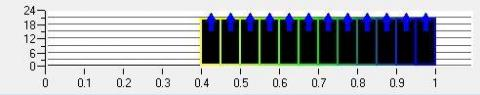Figure 3. Mesh quality of 30°/30° plate

## 4.jpg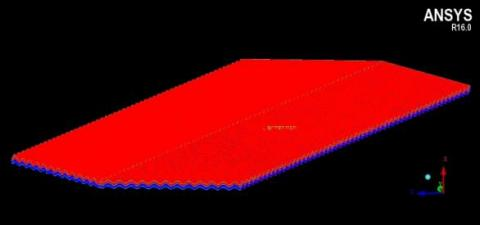Figure 4. Mesh of 60°/60° plate on ICEM CFD Ansys

## 5.jpg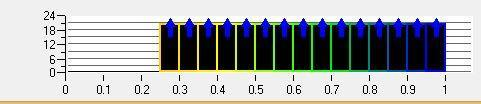Figure 5. Mesh quality of 60°/60° plate

Then we assumed the initial temperature of hot fluid such that the average of inlet and outlet temperature becomes equal to the required temperature. Several iterations were required to determine the correct inlet temperature and velocity.

3.3 Simulations and recording of results

After the setting of the parameters, the ANSYS Solver Manger was opened and the required case file was loaded. The simulations were set to run in local parallel using 4 partitions. All simulations of different inlet conditions were carried out in the same way. The results of simulations were recorded using ANSYS CFX Post. The values of inlet pressure, outlet pressure, outlet temperature and mass flow rate were determined for both the hot as well as the cold fluid. The temperature contour and streamlines of 30°/30° configuration is shown in Figure 6 and Figure 7, respectively. While for 60°/60° configuration, the temperature contour and streamlines are shown in Figure 8 and Figure 9, respectively.

## 6.jpg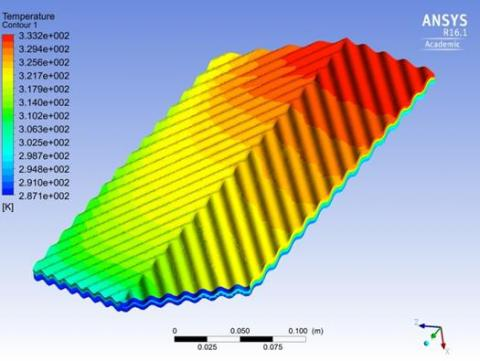Figure 6. Temperature contour of 30°/30° plate at Re = 500,  Pr = 3.5

## 7.jpg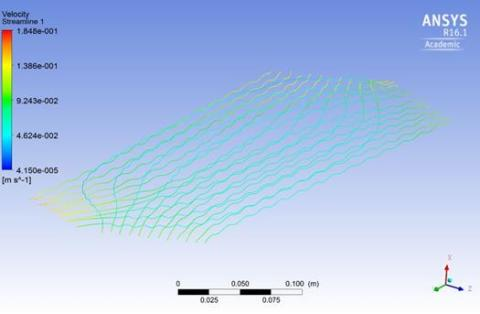Figure 7. Streamlines of 30°/30° plate at Re = 500, Pr = 3.5

## 8.jpg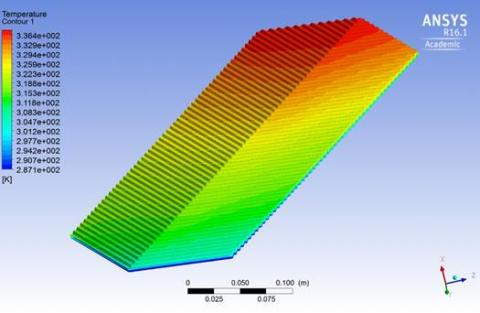Figure 8. Temperature contour of 60°/60° plate at Re = 500, Pr = 3.5

## 9.jpg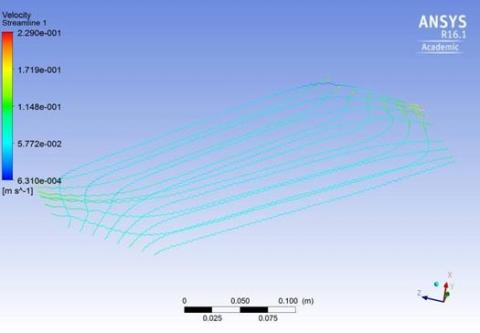Figure 9. Streamlines of 60°/60° plate at Re = 500, Pr = 3.5

4. Data Reduction

The data is reduced to obtain the required correlations of Nusselt number and Reynolds number.

4.1 Modified Wilson plot method

This method is used to obtain the multiplier and exponent of Reynolds number in the following generalized Nusselt number correlation

$Nu=CRe^m Pr^{n.}(μ/μ_s)^{0.14}$          (1)

C, m and n are independent of the nature of fluid used.

The heat transfer coefficients for the cold and hot sides of PHE are obtained by the following equations, respectively

$h_{c=k_c/(D_{hyd})} C_c Re_c^p Pr_c^{1/3}(μ/μ_s )^{.13}$          (2)

$h_{h=k_c/(D_{hyd})} C_h Re_c^p Pr_h^{1/3}(μ/μ_s )^{.13}$          (3)

These two results are then plugged in the following equation

$1/U=1/h_c +(t/k)_w+1/h_h$          (4)

By solving, we obtained the following equations

$1/U-(t/k)_w=1/(C_c k_c/D_{hyd} Re_c^p Pr_c^{1/3} (μ/μ_s )^{.14}+1/(C_h k_h/D_{hyd} Re_h^p Pr_h^{1/3} (μ/μ_s ){^.14}$          (5)

$(1/U-(t/k)_{wall})[k_h/D_{hyd}((ρvD_{hyd})/μ)_h^p ((C_p μ)/k)_h^{1⁄3} (μ/μ_s )_h^{0.14}]=1/C_h +(k_h/D_{hyd} ((ρvD_{hyd})/μ)_h^p ((C_p μ)/k)_h^(1⁄3) (μ/μ_s )_h^{0.14}/(C_h k_c/D_{hyd} ((ρvD_{hyd})/μ)_c^p ((C_p μ)/k)_c^{1⁄3} (μ/μ_s )_c^{0.14} )$          (6)

This equation is then compared to the general equation of slope

$Y_1=mX_1+b$        (7)

where

$Y1=(1/U-(t/k)_{wall})[k_h/D_{hyd} ((ρvD_{hyd})/μ)_h^p ((C_p μ)/k)_h^{1⁄3} (μ/μ_s )_h^{0.14}]$           (8)

$X1=(k_h/D_{hyd} ((ρvD_{hyd})/μ)_h^p ((C_p μ)/k)_h^{1⁄3} (μ/μ_s )_h^{0.14})/(C_h k_c/D_{hyd} ((ρvD_{hyd})/μ)_c^p ((C_p μ)/k)_c^{1⁄3} (μ/μ_s )_c^{0.14} )$          (9)

slope:     $m=1/C_h$

intercept:    $b=1/C_c$

X1 & Y1 are calculated from the data obtained from simulations and value of exponent ‘p’ is assumed initially. Using X1 and Y1, the plot is made and values of slope (m) and intercept (b) can be determined.

4.1.1 Logarithmic modification

The logarithmic modification of equation (6) is given below:

$(1/U-(t/k)_{wall})= 1/(C_h k_h/D_{hyd} Re_h^p Pr_h^{1⁄3} (μ/μ_s )_h^{0.14})+1/(C_c k_c/D_{hyd} Re_c^p Pr_c^{1⁄3} (μ/μ_s )_c^{0.14})$        (10)

$(1/U-t/k-1/[C_c Re_c^p Pr _c^{1⁄3} (μ/μ_s )_c^{0.14} K_c/D_h ] ) Pr_h^{1⁄3} (μ/μ_s )_c^{0.14} K_c/D_h =1/((C_h Re_h^p ) )$        (11)

$X2=ln⁡(Re_h)$           (12)

$y_2=1/((C_h Re_h^p ) )$          (13)

$Y2=lny_2=-lnC_h-pln(Re_h )$         (14)

4.1.2 Iterative procedure

Procedure for finding the values of ‘p’ and ‘Ch’ is given below:

1. An initial value of ‘p’ is assumed and put in X1,Y1 plot

2. The plot of equation 2 & 3 will give us values of ‘Ch’ and ‘Cc’.

3. Plug in these values of ‘Ch’ and ‘Cc’ and plot X2, Y2.

4. Plot of X2 and Y2 will yield new value of p.

5. Repeat this iteration until the difference of the previous value and the present value becomes ≤0.02 which is our.

5. Results

Using the simulation results and Modified Wilson, the constant multiplier (C) and the exponent (p) of Re are determined for each of the plate configuration data. The Prandtl Number (Pr) exponent is taken to be 1/3 because literature reveals that its value tends to remain between 0.3 and 0.4. This effect is further investigated below. The classical value of 0.14 is used for the exponent of (μ/μs).

5.1 Modified Wilson plots

For 30/30 configuration, the Wilson plot of X1 vs. Y1 at Pr=7.5, is shown in Figure 10, while plot of X2 vs. Y2 at pr=7.5 is shown in Figure 11. The slope of X2 vs. Y2 gives the reciprocal of exponent of the Reynolds number and the intercept gives the value of reciprocal of Ch. Likewise, for 60/60 configurations, the Wilson plot of X1 vs Y1, and X2 vs. Y2 at Pr value of 4.5 is shown in Figure 12 and Figure 13, respectively.

5.2 Nusselt number correlation

The following correlations have been developed using the value of ‘p’ and ‘c’ obtained from the above graphs.

$Nu=0.093R e^{0.7106} Pr^{1.3} (μ/μ_s)^{0.14}$    β= 30°/30°       (15)

$Nu=0.112R e^{0.714} Pr^{1.3} (μ/μ_s)^{0.14}$    β=60°/60°          (16)

## 10.jpg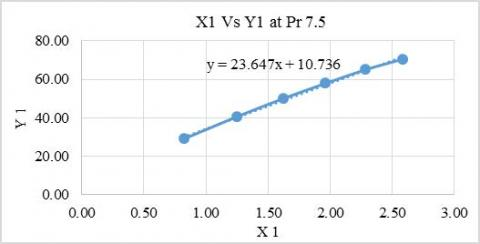Figure 10. Linear Plot for 30/30 plate at Pr = 7.5

## 11.jpg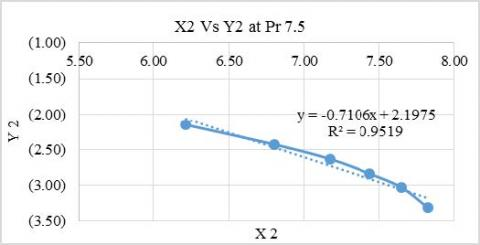Figure 11. Logarithmic plot for 30/30 plate at Pr = 7.5

## 12.jpg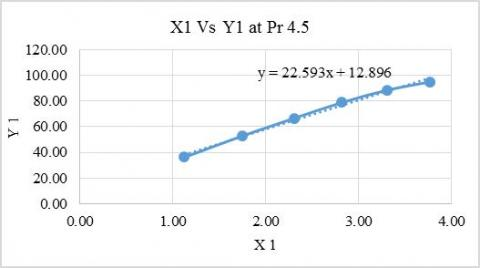Figure 12. Linear plot for 60/60 plate at Pr = 4.5

## 13.jpg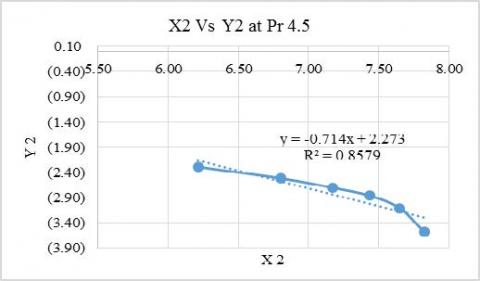Figure 13. Logarithmic Plot for 60/60 plate at Pr = 4.5

6. Conclusions

The simulation was carried out to develop the generalized Nusselt number correlations for a commercial plate heat exchanger configuration using water as the working fluid for both hot and cold sides. We chose hot side fluid as our reference fluid, therefore only behaviour of hot side fluid to changing conditions was observed. Hot side Nusselt number was found to increase with increasing Reynolds number as well as by increasing the chevron angle. Prandtl number, however, does not affect the Nusselt number by a considerable amount. Since it depends mainly on temperature i.e. higher the average temperature, lower will be the Prandtl number, therefore working on lower Prandtl number is advisable as it yields better heat transfer result. Our presented correlations are valid for 500<Re<2500 and 3.5<Pr<7.5.

Nomenclature

A      Surface area for heat transfer, m2

Ax     Channel flow area, m2

b       Mean channel spacing, m

Cp     Specific heat capacity, J/kg.K

dx     Wall thickness, m

h       Individual convection heat transfer of fluid on each side, W/m2.K

k       Thermal conductivity

kf      Thermal conductivity of fluid, W/m.K

L       Characteristic length, m

LMTD      Log Mean Temperature Difference

m      Mass flow rate, kg/s

Pr     Prandtl number

q      Rate of heat transfer, W

Re    Reynolds number

t        Plate thickness, m

T       Temperature, K

U      Overall heat transfer coefficient, W/m2.K

V      Mean velocity of fluid, m/s

Greek symbols

β       Chevron angle, degree

µ       Dynamic viscosity, N.s/m2

ƿ       Density of fluid, kg/m3

Φ      Surface enlargement factor

Subscripts

c       Cold

h       Hot

i        Inlet

o       Outlet

w      Wall

avg   Average

References

 Shah R.K., Sekulic D.P. (2003). Fundamentals of heat exchanger design, John Wiley & Sons, USA.

 Fernandez-Seara J., Uhía F.J., Sieres J., Campo A.A. (2007). General review of the Wilson plot method and its modifications to determine convection coefficients in heat exchange devices, Applied Thermal Engineering, Vol. 27, No. 17-18, pp. 2745-2757, DOI: 10.1016/j.applthermaleng.2007.04.004

 Khan T.S., Khan M.S., Chyu M.C., Ayub Z.H. (2010). Experimental investigation of single phase convective heat transfer coefficient in a corrugated plate heat exchanger for multiple plate configurations, Applied Thermal Engineering, Vol. 30, No. 8-9, pp. 1058-1065, DOI: 10.1016/j.applthermaleng.2010.01.021

 Yaïci W., Ghorab M., Entchev E. (2016). 3D CFD study of the effect of inlet air flow maldistribution on plate-fin-tube heat exchanger design and thermal–hydraulic performance, International Journal of Heat and Mass Transfer, Vol. 101, pp. 527-541. DOI: 10.1016/j.ijheatmasstransfer.2016.05.063

 Hashmi A., Tahir F., Hameed U. (2012). Empirical Nusselt number correlation for single phase flow through a plate heat exchanger, Proceedings of the 9th WSEAS International Conference on Heat and Mass Transfer (HMT’12), Cambridge, MA, USA, pp. 25-27.

 Muley A., Manglik R. (1999). Experimental study of turbulent flow heat transfer and pressure drop in a plate heat exchanger with chevron plates, Journal of heat transfer, Vol. 121, No. 1, pp. 110-117. DOI: 10.1115/1.2825923.

 Akturk F., Gulben G., Aradag S., Uzol N.S., Kakac S. (2011). Experimental investigation of the characteristics of a chevron type gasketed-plate heat exchanger, International Advanced Technologies Symposium (IATS’11), pp. 16-18.

 Turk C., Aradag S., Kakac S. (2016). Experimental analysis of a mixed-plate gasketed plate heat exchanger and artificial neural net estimations of the performance as an alternative to classical correlations, International Journal of Thermal Sciences, Vol. 109, pp. 263-269. DOI: 10.1016/j.ijthermalsci.2016.06.016

 Quintero A.E.,Vera M. (2017). Laminar counterflow parallel-plate heat exchangers: an exact solution including axial and transverse wall conduction effects, International Journal of Heat and Mass Transfer, Vol. 104, pp. 1229-1245. DOI: 10.1016/j.ijheatmasstransfer.2016.09.025.

 Kumar B., Singh S.N. (2015). Analytical studies on the hydraulic performance of chevron type plate heat exchanger, International Journal of Heat and Technology, Vol. 33, No. 1, pp. 17-24. DOI: 10.18280/ijht.330103

 Hazmi A.S.A., Maurad Z.A., Pauzi N.N.P.N., Idris Z. (2016). Rapid evaluation of plate heat exchanger performance and fouling analysis in epoxidation of oleochemical at pilot plant scale, International Journal of Heat and Technology, Vol. 34, No. 4, pp. 558-564. DOI: 10.18280/ijht.340402

 Doohan R.S., Kush P.K., Maheshwari G. (2016). Exergy based optimization and experimental evaluation of plate fin heat exchanger, Applied Thermal Engineering, Vol. 102, pp. 80-90. DOI: 10.1016/j.applthermaleng.2016.03.101

 Zhang Y., Jiang C., Yang Z., Zhang Y., Bai B. (2016). Numerical study on heat transfer enhancement in capsule-type plate heat exchangers, Applied Thermal Engineering, Vol. 108, pp. 1237-1242. DOI: 10.1016/j.applthermaleng.2016.08.033

 Khan T.S. (2011). Evaporation in flooded corrugated plate heat exchanger with ammonia and ammonia missible oil, Ph.D. dissertation, Ghulam Ishaq Khan Institute of Engineering Sciences & Technology, Swabi.